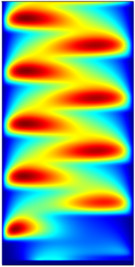# Application Gallery

## Dielectric Barrier Discharge

Application ID: 8637

This model simulates electrical breakdown in an atmospheric pressure gas. Modeling dielectric barrier discharges in more than one dimension is possible, but the results can be difficult to interpret because of the amount of competing physics in the problem.

In this simple model the problem is reduced to 1D by assuming the dielectric gap is much smaller than the diameter of the plates. To highlight the physics of the breakdown process, the model uses a simple argon chemistry, which keeps the number of species and reactions to a minimum.This model example illustrates applications of this type that would nominally be built using the following products: